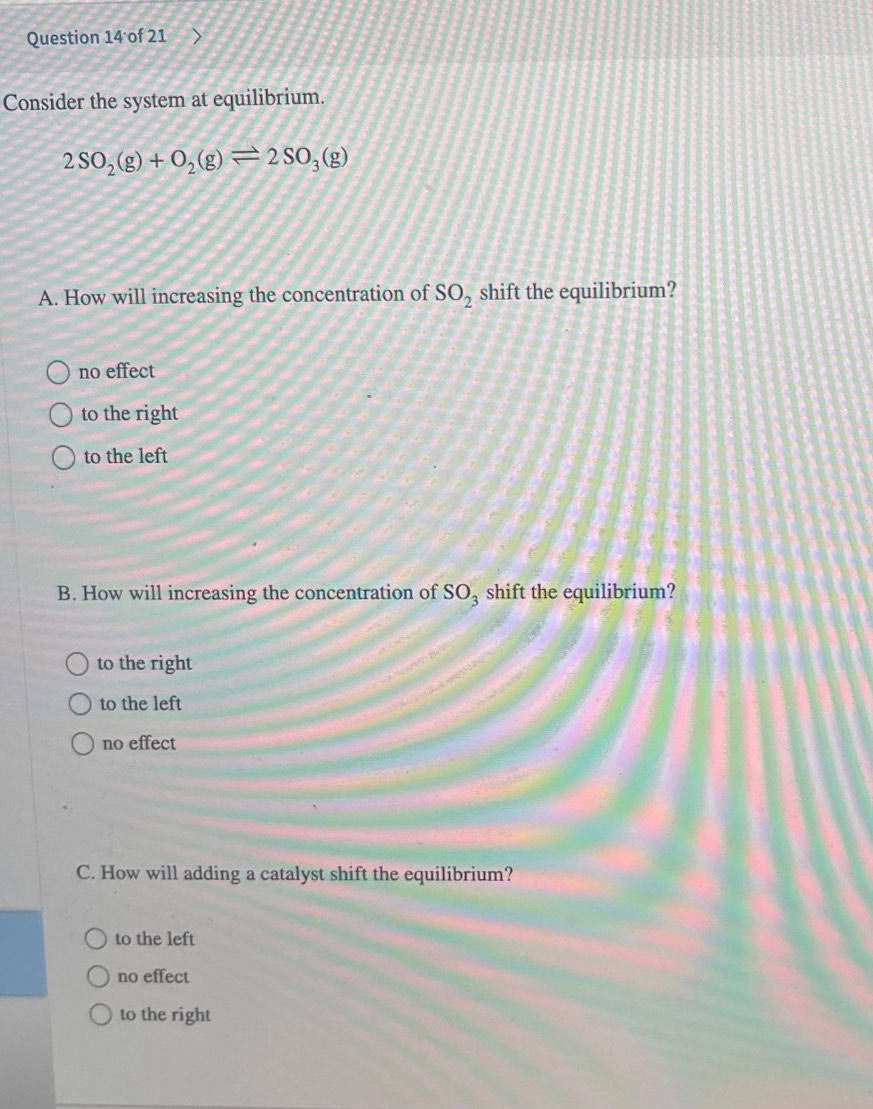Question:

# Consider the system at equilibrium. 2SO₂(g) + O₂(g)N₂(g) ⥨2 SO₂(g) A. How will increasing the concentration of SO₂ shift the equilibrium? ○ no effect ○ to the right ○ to the left B. How will increasinConsider the system at equilibrium. 2SO₂(g) + O₂(g)N₂(g) ⥨2 SO₂(g) A. How will increasing the concentration of SO₂ shift the equilibrium? ○ no effect ○ to the right ○ to the left B. How will increasing the concentration of SO3 shift the equilibrium? ○ to the right ○ to the left ○ no effect C. How will ding a catalyst shift the equilibrium? ○ to the left ○ no effect O to the right